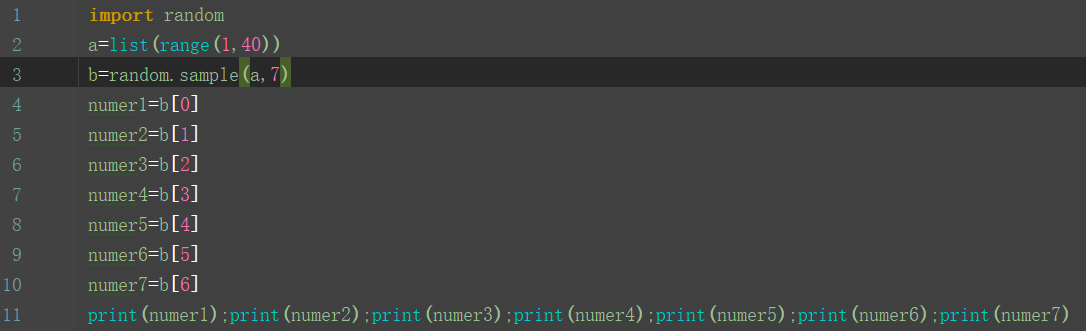3个回答

import random
a = list(range(1,40))
index = 22 #指定不被抽到的随机数
flag = True
while flag:

print(b[0:7])

c = [ x,y,z]
b= random.sample(a,7)
if not b in c:
pass